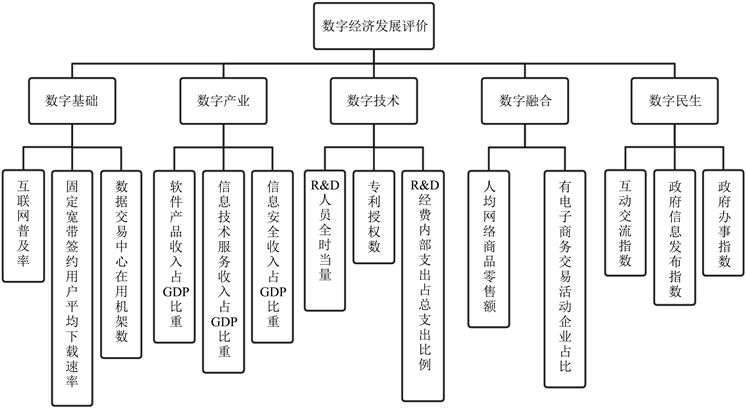﻿ 基于层次分析法的数字经济发展综合评价——以长江三角洲为例

# 基于层次分析法的数字经济发展综合评价——以长江三角洲为例Comprehensive Evaluation of Digital Economy Development Based on Analytic Hierarchy Process—A Case Study of the Yangtze River Delta Region

Abstract: As a new economic form, digital economy is not only an important driving force for economic growth, but also the core of enhancing national economic vitality and competitiveness. Therefore, scientific evaluation of the development of digital economy is conducive to promoting the healthy development of digital economy. Based on the study of digital economy, this paper establishes the evaluation system of digital economy from five aspects, uses the analytic hierarchy process (AHP) to assign weights to indicators, and uses dimensionless to make score statistics on the digital economy development index data of provinces (municipalities) in the Yangtze River Delta in 2019. It is found that Jiangsu province has the best digital economy development, Anhui province is lagging behind, and the Yangtze River Delta digital economy development is unbalanced. Accordingly, relevant suggestions are given: Laying a good foundation for the development of digital economy; Attaching importance to the development of digital industrialization; Increasing the input of digital technology talents and funds and strengthening the infiltration from Jiangsu, Zhejiang and Shanghai to Anhui.

1. 引言

2. 数字经济的内涵

3. 数字经济的评价体系

4. 数字经济发展评价体系设计

4.1. 指标选取及数据来源Table 1. Evaluation index of digital economy development

4.2. 权重确定

4.2.1. 构造判断矩阵和计算权重向量Figure 1. Evaluation index of digital economy developmentTable 2. Index relative importance hierarchy

4.2.2. 层析排序权重及判断矩阵的一致性检验

${W}_{i}=\sqrt[n]{{M}_{i}},\text{\hspace{0.17em}}\text{\hspace{0.17em}}i=1,2,3,\cdots ,n$ (1)

${W}_{i}=\frac{{\stackrel{¯}{W}}_{i}}{{\sum }_{i=1}^{n}{\stackrel{¯}{W}}_{i}},\text{\hspace{0.17em}}\text{\hspace{0.17em}}i=1,2,3,\cdots ,n$ (2)

$CI=\frac{{\lambda }_{\mathrm{max}}-n}{n-1}$ (3)Table 3. Mean random consistency

$CR=\frac{CI}{RI}$ (4)

$\begin{array}{c}CR=\frac{{\omega }_{A1}C{I}_{\text{1}}+{\omega }_{A2}C{I}_{2}\text{+}{\omega }_{A\text{3}}C{I}_{3}\text{+}{\omega }_{A\text{4}}C{I}_{4}}{{\omega }_{A1}R{I}_{\text{1}}+{\omega }_{A2}R{I}_{2}+{\omega }_{A3}R{I}_{3}+{\omega }_{A4}R{I}_{4}}\\ =\frac{{\omega }_{A1}C{I}_{\text{1}}+{\omega }_{A2}C{I}_{2}}{{\omega }_{A1}R{I}_{\text{1}}+{\omega }_{A2}R{I}_{2}}\end{array}$ (5)

$CR<0.1$，则判别矩阵通过一致性检验

4.3. 无量纲化

1) 确定评价对象的因素领域 $U=\left\{{u}_{1},{u}_{2},\cdots ,{u}_{m}\right\}$

2) 确定评语等级论域 $V=\left\{{v}_{1},{v}_{2},\cdots {v}_{n}\right\}$

3) 进行单因素评价，建立模糊关系矩阵 $R=\left(\begin{array}{ccc}{r}_{11}& \cdots & {r}_{1n}\\ ⋮& \ddots & ⋮\\ {r}_{m1}& \cdots & {r}_{mn}\end{array}\right)$

4) 确定评价因素的模糊权向量 ${g}_{i}$

5) 模糊综合评价 $H=G×R=\left({g}_{1},{g}_{2},\cdots {g}_{m}\right)\left(\begin{array}{ccc}{r}_{11}& \cdots & {r}_{1n}\\ ⋮& \ddots & ⋮\\ {r}_{m1}& \cdots & {r}_{mn}\end{array}\right)=\left({h}_{1},{h}_{2},\cdots ,{h}_{m}\right)$

6) 无量纲化处理：正向指标 ${d}_{i}=\frac{{h}_{i}}{\mathrm{min}\left\{{h}_{i}|i=1,2,\cdots ,m\right\}}\left(i=1,2,\cdots ,m\right)$ 负向指标 ${d}_{i}=\frac{\mathrm{min}\left\{{h}_{i}|i=1,2,\cdots ,m\right\}}{{h}_{i}}$ $\left(i=1,2,\cdots ,m\right)$Table 4. Yangtze River Delta provinces (municipalities) each index score

5. 政策建议

1) 打好数字经济发展基础

2) 重视数字产业化发展

“十四五”规划提出要加快数字化发展，打造具有国际竞争力的数字产业集群。浙江省和安徽省数字产业GDP占比较低，从数字产业的权重可以看出数字产业在数字经济发展中的核心地位，数字产业是数字经济可量化衡量的最重要方面，数字产业的GDP占比反映了数字经济对经济总量的带动作用，数字产业GDP占比越大，数字经济越活跃。上海市数字产业在数字基础的建设中，尤其在2019年与中国联通、中国电信先后签署5G战略合作框架协议后，催生了一大批企业进入数字产业领域并不断拓展新的增长空间。

3) 加大数字技术人才和经费的投入

4) 发挥江浙沪带头作用，加强江浙沪向安徽省的渗透力，促进长江三角洲数字经济协调发展

 方晓霞, 杨丹辉, 李晓华. 新科技革命与产业革命深度交互下超智慧社会构建——日本的战略演进与机制创新[J]. 日本问题研究, 2018, 32(1): 11-20.

 尹丽波. 数字经济引领中国转型发展. 载, 马化腾, 孟昭莉, 闫德利, 等. 数字经济——中国创新增长新动能[M]. 北京: 中信出版社, 2017: XVI.

 耿德林. 长江三角洲地区金融创新、金融集聚与经济协调发展研究[J]. 征信, 2020, 38(12): 83-88.

 崔保国, 刘金河. 论数字经济的定义与测算——兼论数字经济与数字传媒的关系[J]. 现代传播(中国传媒大学学报), 2020, 42(4): 120-127.

 UNCTAD (2019) Digital Economy Report 2019. United Nations, Geneva.

 Tapscott, D. (1994) The Digital Economy: Promise and Peril in The Age of Networked Intelligence. McGraw-Hill, New York, xiii.

 中国信息通信研究院. 《中国数字经济发展白皮书(2017年)》[EB/OL]. http://www.cac.gov.cn/2017-07/13/c_1121534346.htm, 2017-07-13.

 邬贺铨. 互联网的新机遇数字经济新动能[J]. 互联网天地, 2017(1): 6-10.

 美国商务部. 浮现中的数字经济[M]. 北京: 中国人民大学出版社, 1998.

 托拜厄斯•阿德里安, 托马索•曼奇尼-格里福, 姜开锋. 数字货币的兴起[J]. 金融市场研究, 2019(9): 39-65.

 du Rausas, M.P., et al. (2011) Internet Matters: The Net’s Sweeping Impact on Growth, Jobs, and Prosperity. Mckinsey Global Institute, New York.

 陆镜弛, 余建坤, 刘明瑶. 数字经济发展评价研究——以云南省为例[J]. 当代经济, 2021(2): 60-65.

Top